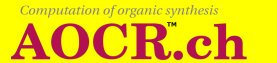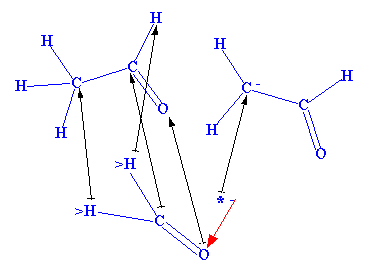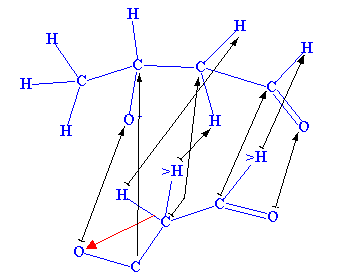# 1. Organic chemistry basics

### 1.3. Reaction step rules

Two matching correspondence for the application of the rules in Figure 1.4 and 1.5 are represented in Figure 1.6 and 1.7. The application of the rule in Figure 1.6 determines the first step of the example reaction in Figure 1.3.

Figure 1.6The application of the rule in Figure 1.7 determines the second step of the example reaction in Figure 1.3.

Figure 1.7